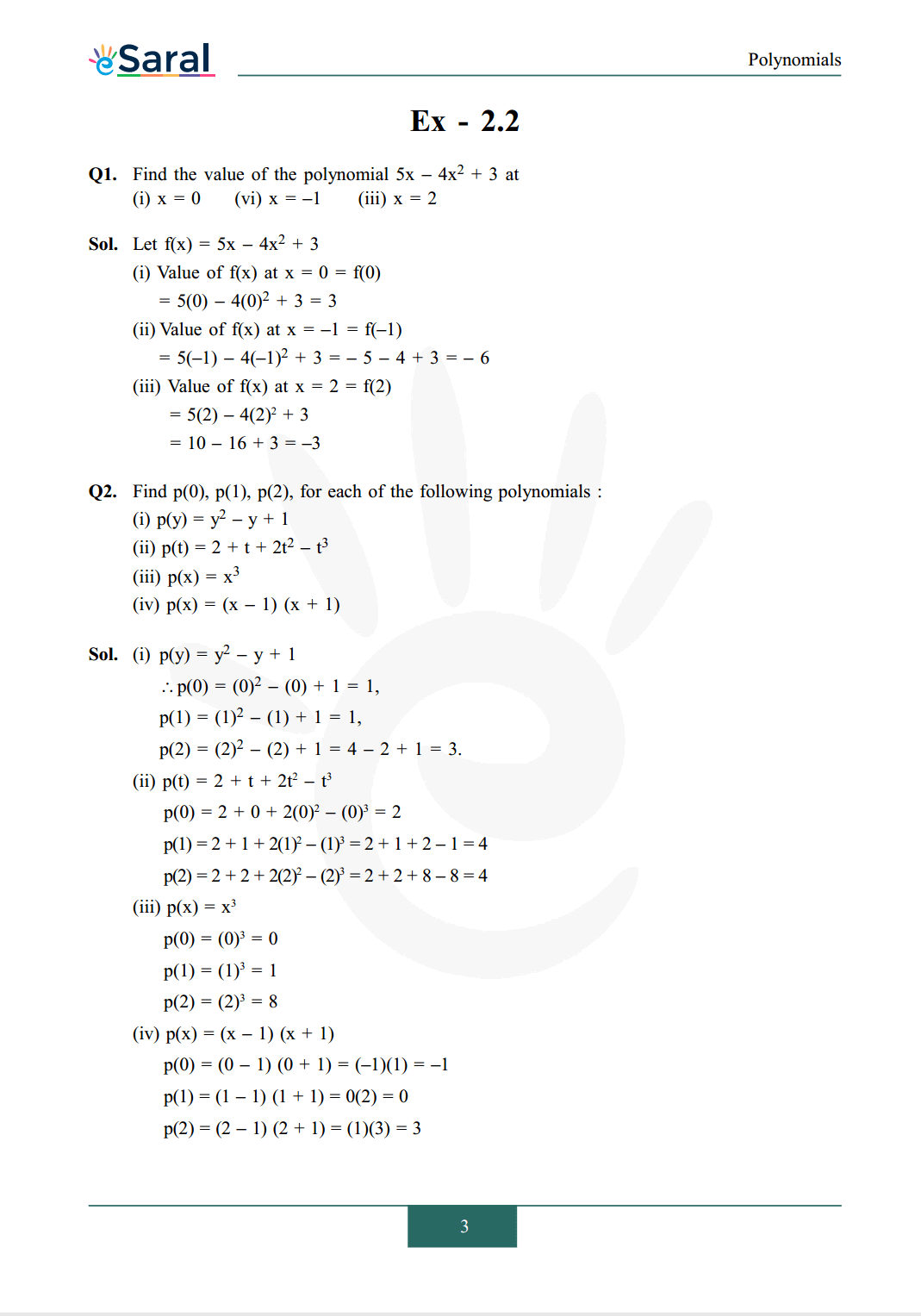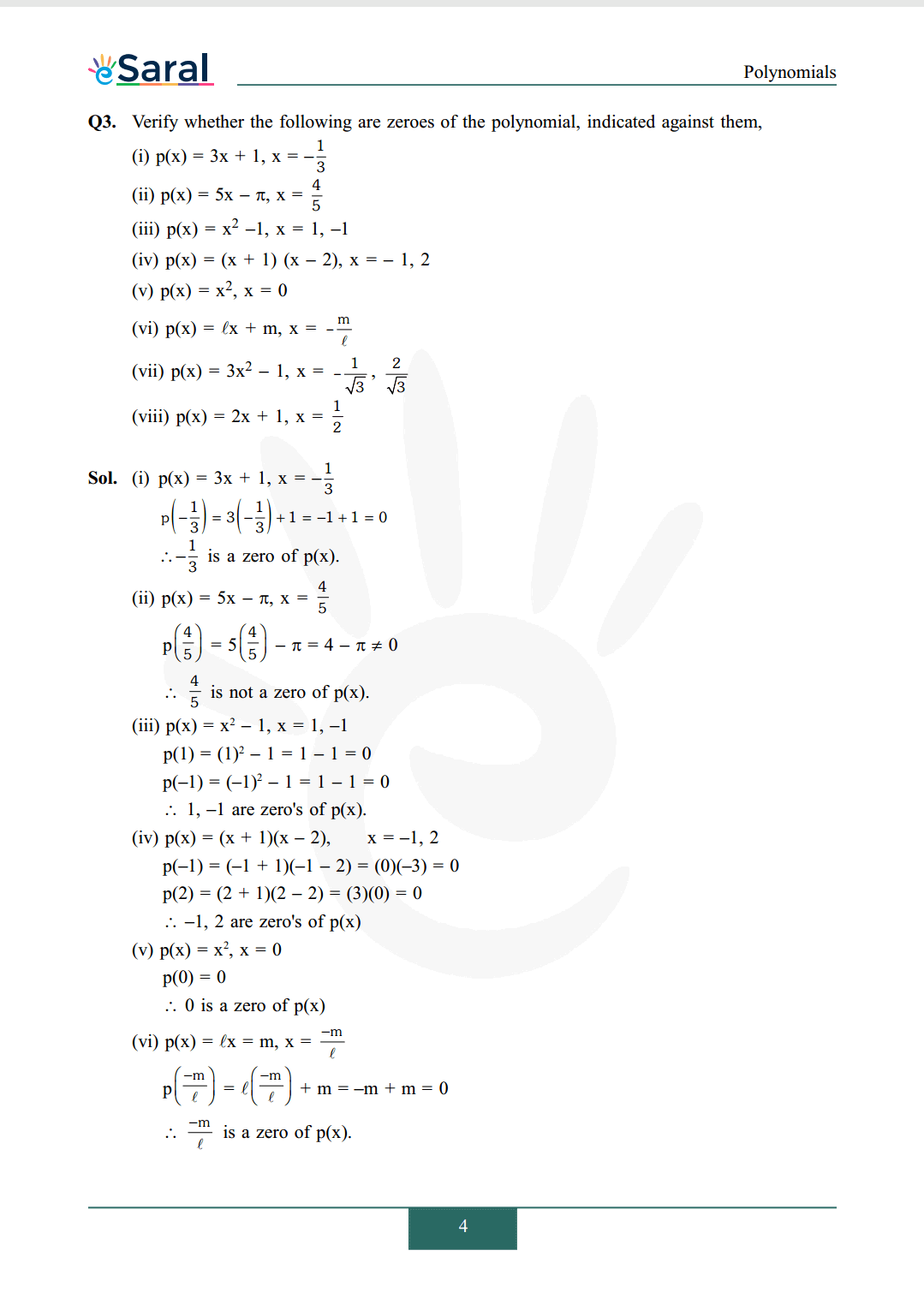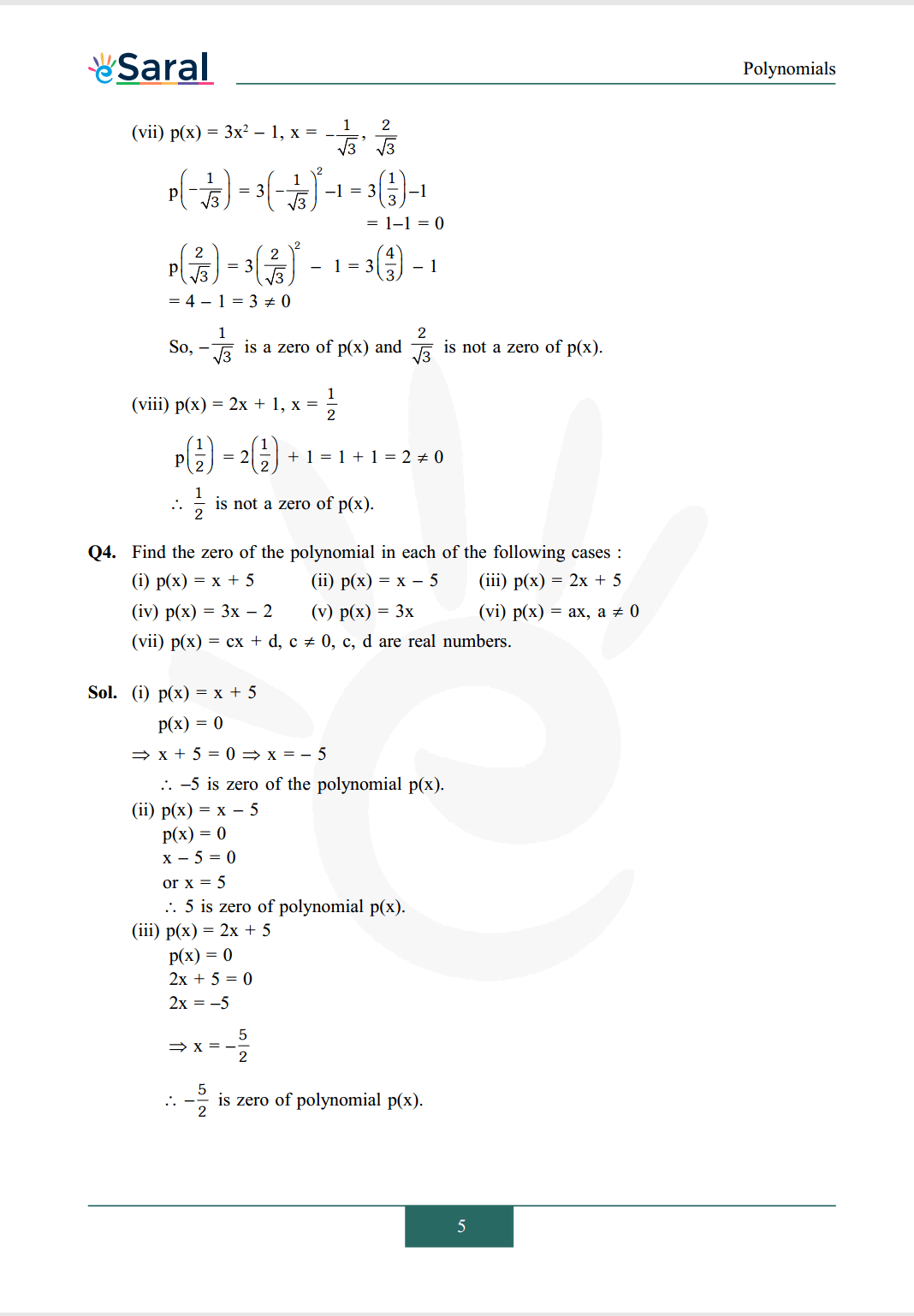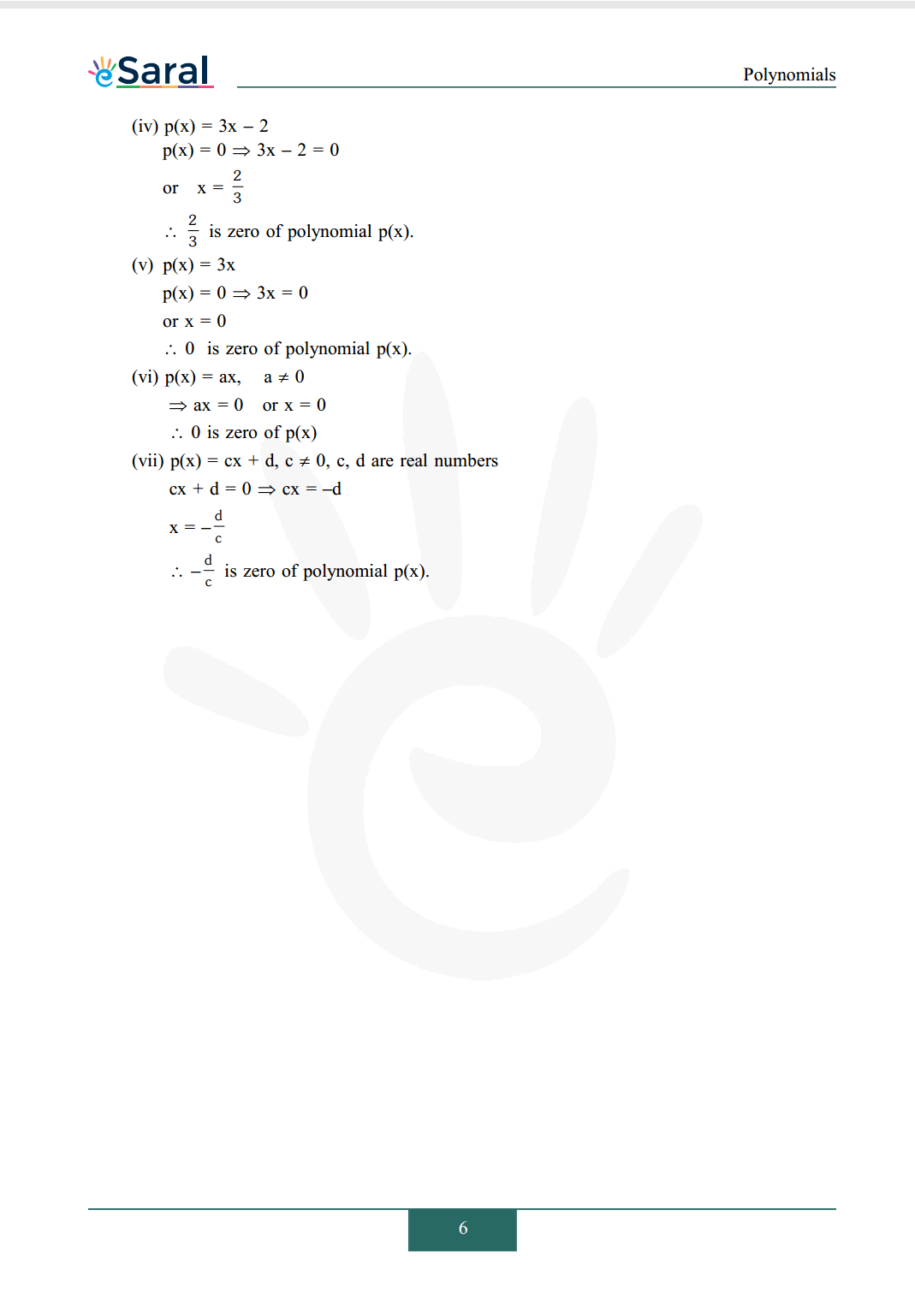Download the eSaral app and start learning from Kota's top IITians and doctors.

# NCERT Solutions for Class 9 Maths chapter 2 Exercise 2.2 - Polynomials - Free PDF DownloadHey, are you a class 9 Student and Looking for Ways to Download NCERT Solutions for Class 9 Maths chapter 2 Exercise 2.2? If Yes then you are at the right place.

Here we have listed Class 9 maths chapter 2 exercise 2.2 solutions in PDF that is prepared by Kota’s top IITian’s Faculties by keeping Simplicity in mind.

If you want to score high in your class 9 Maths Exam then it is very important for you to have a good knowledge of all the important topics, so to learn and practice those topics you can use eSaral NCERT Solutions.

So, without wasting more time Let’s start.

### Download The PDF of NCERT Solutions for Class 9 Maths chapter 2 Exercise 2.2 "Polynomials"#### All Questions of Chapter 2 Exercise 2.2

Once you complete the chapter 2 then you can revise Ex. 2.2 by solving following questions

Q1. Find the value of the polynomial $5 x-4 x^{2}+3$ at
(i) $\mathrm{x}=0$
(vi) $\mathrm{x}=-1$
(iii) $\mathrm{x}=2$

Q2. Find $\mathrm{p}(0), \mathrm{p}(1), \mathrm{p}(2)$, for each of the following polynomials :
(i) $p(y)=y^{2}-y+1$
(ii) $\mathrm{p}(\mathrm{t})=2+\mathrm{t}+2 \mathrm{t}^{2}-\mathrm{t}^{3}$
(iii) $\mathrm{p}(\mathrm{x})=\mathrm{x}^{3}$
(iv) $\mathrm{p}(\mathrm{x})=(\mathrm{x}-1)(\mathrm{x}+1)$

Q3. Verify whether the following are zeroes of the polynomial, indicated against them,
(i) $\mathrm{p}(\mathrm{x})=3 \mathrm{x}+1, \mathrm{x}=-\frac{1}{3}$
(ii) $\mathrm{p}(\mathrm{x})=5 \mathrm{x}-\pi, \mathrm{x}=\frac{4}{5}$
(iii) $\mathrm{p}(\mathrm{x})=\mathrm{x}^{2}-1, \mathrm{x}=1,-1$
(iv) $\mathrm{p}(\mathrm{x})=(\mathrm{x}+1)(\mathrm{x}-2), \mathrm{x}=-1,2$
(v) $\mathrm{p}(\mathrm{x})=\mathrm{x}^{2}, \mathrm{x}=0$
(vi) $\mathrm{p}(\mathrm{x})=\ell \mathrm{x}+\mathrm{m}, \mathrm{x}=-\frac{\mathrm{m}}{\ell}$
(vii) $\mathrm{p}(\mathrm{x})=3 \mathrm{x}^{2}-1, \mathrm{x}=-\frac{1}{\sqrt{3}}, \frac{2}{\sqrt{3}}$
(viii) $\mathrm{p}(\mathrm{x})=2 \mathrm{x}+1, \mathrm{x}=\frac{1}{2}$

Q4. Find the zero of the polynomial in each of the following cases:
(i) $p(x)=x+5$
(ii) $\mathrm{p}(\mathrm{x})=\mathrm{x}-5$
(iii) $\mathrm{p}(\mathrm{x})=2 \mathrm{x}+5$
(iv) $\mathrm{p}(\mathrm{x})=3 \mathrm{x}-2$
(v) $\mathrm{p}(\mathrm{x})=3 \mathrm{x}$
(vi) $\mathrm{p}(\mathrm{x})=\mathrm{ax}, \mathrm{a} \neq 0$
(vii) $\mathrm{p}(\mathrm{x})=\mathrm{cx}+\mathrm{d}, \mathrm{c} \neq 0, \mathrm{c}, \mathrm{d}$ are real numbers.

Class 9 Maths Chapter 1 Exercise 1.1 Solutions

Class 9 Maths Chapter 1 Exercise 1.2 Solutions

Class 9 Maths Chapter 1 Exercise 1.3 Solutions

If you have any Confusion related to NCERT Solutions for Class 9 Maths chapter 2 Exercise 2.2 then feel free to ask in the comments section down below.

To watch Free Learning Videos on Class 9 by Kota’s top Faculties Install the eSaral App

Mahi
May 12, 2023, 6:35 a.m.
Best
Mahi
May 12, 2023, 6:35 a.m.
Best
Mahi
May 12, 2023, 6:35 a.m.
Best
Mahi
May 12, 2023, 6:35 a.m.
Best
PRIYANSHI MARSKOLE
Sept. 10, 2021, 9:56 p.m.
Que.3 (x+1) (x-2) ,x=-1,2 Solution.